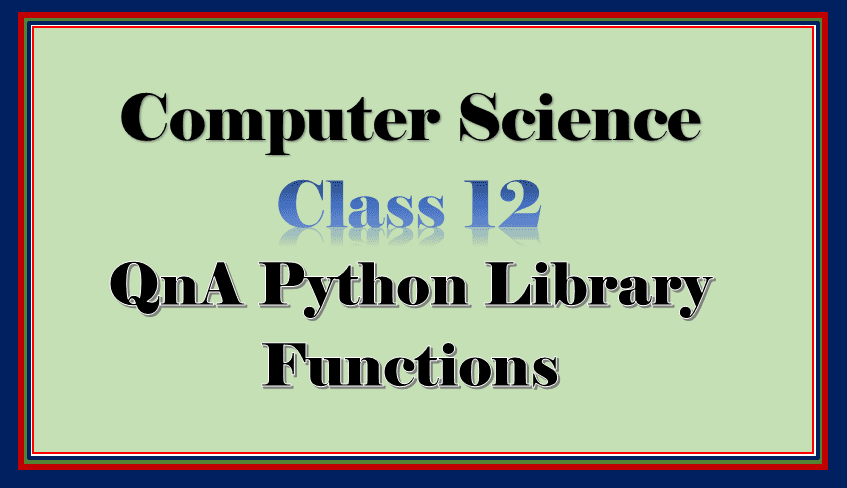Important QnA python library functions 12 provides you certain questions and answers for CBSE class 12 Computer Science subject. So here we begin!

## Important QnA python library functions 12

Follow the below given link for answers which are not available below:

Q – 1 What do you mean by Library Function in Python?

Q – 2 Enlist some commonly used libraries of python and explain in short.

Q – 3 Explain the structure of python module.

Q – 4 How to import a python module? or What are the ways to insert python modules in a program?

Ans.: To insert a python module you can use the import statement in the following two ways:

1. import command: Ex. import math
2. from import: Ex. from math import *

Q – 5 Explain some commonly used mathematical functions in detail with example.

Q – 6 Explain some commonly used string functions in detail with example.

Q – 7 Write related library function name to do the following:

1. Display the negative number into positive: abs()
2. Display nearest integer for the given number: ceil()
3. Get the remainder: fmod(), remainder()
4. Get the compute cube of a given number: pow()
5. Get the square root of the given number: sqrt()
6. Convert the first letter of the text into capital: capitalise()
7. Join two words: join()
8. Convert the text into the lower case: lower()

Q – 8 Name the module required to import for the following functions:

1. pow() – math
2. fabs() – math

In the next section of important QnA python library functions 12 you will find some output questions.

Q – 9 Find the output for the following:

1.

``````def str_exp():
str1=str1.lower()
str2 =str1.split(',')
for i in str2:
if i<'s':
print(str.lower(i))
else:
print(str.upper(i))
str_exp()``````

covid-19

SANITIZER

lockdown

In the above example, the text starts with the alphabet ‘s’ and letter coming after ‘s’ will be converted into uppercase and rest text will be in lower case.

2.

``````def str_exp():
str1='Covid - 19 forces the entire worl to be lockdown'
str1=str1.replace('e','i')
print(str1)
str_exp()``````

Covid – 19 forcis thi intiri worl to bi lockdown

All e replace with the letter i in the text.

3.

``````def str_exp():
str="Lockdown to Unlock 1.0"
d=str.split()
print(d)
str_exp()``````

[‘Lockdown’, ‘to’, ‘Unlock’, ‘1.0’]

The words will be separated in a string after space

4.

``````import math
def mth_exp():
a=5.5
b=3.75
c=2.25
print(round(math.fsum([a,b,c]),0))
mth_exp()``````

12.0

Function fsum() returns sum of specified variables a,b,c. The values are 5.5 + 3.75 + 2.25 = 11.5. Then round function returns next integer if the adjecent digit is more than 5. So the final output will be 12.0

In the next section of important QnA python library functions 12 we will provide you some programs for QnA python library functions 12.

1. Write a program to accept the marks of 5 subjects and do the following:

1. Display the total using fsum() function
2. Display total marks in round integers

Ans.:

``````import math
def compute_result():
eng=float(input("Enter marks of English:"))
phy=float(input("Enter marks of Physics:"))
che=float(input("Enter marks of Chemistry:"))
mat=float(input("Enter marks of Maths:"))
cs=float(input("Enter marks of Computer Science:"))
tot=round(math.fsum([eng,phy,che,mat,cs]),0)
print("Total:",tot)
compute_result()``````

2. Write a program to display the computation of power using math module function.

Ans.:

``````import math
def compute_power():
no=int(input("Enter the number:"))
p=int(input("Enter the power to be raised:"))
ans=math.pow(no,p)
print("The",p,"power of ",no," is:", ans)
compute_power()``````

3. Write a program to convert the first letter of the sentence into a capital letter.

Ans.:

``````def first_upper():
s=input("Enter the sentences:(in lower case:)")
print(s.capitalize())
first_upper()``````

4. Write a program to convert the first letter of each word of the sentence into a capital letter.

``````def first_upper():
s=input("Enter the sentences:(in lower case:)")
print(s.title())
first_upper()``````

So I hope you enjoyed this article the article important QnA python library functions 12. Hit the like button and share it with your friends. Leave your doubts and queries for us in the comment section. Share your feedback, suggestions, views in the comment section as well.

Thank you for visiting our blog.

Follow this link for related article to QnA python library functions 12.